# 1-Propanol (P°i = 20.9 Torr at 25 °C) and 2-propanol (P2 = 45.2 Torr at 25 °C) form ideal solutions in all proportions.Let æ1 and æ2 represent the mole fractions of 1-propanol and 2-propanol in a liquid mixture, respectively, and y, and y2represent the mole fractions of each in the vapor phase.For a solution of these liquids with æ1 = 0.560, calculate the composition of the vapor phase at 25 °C.Y2 =

Question
5 views

I'm not sure how I could answer the question attached.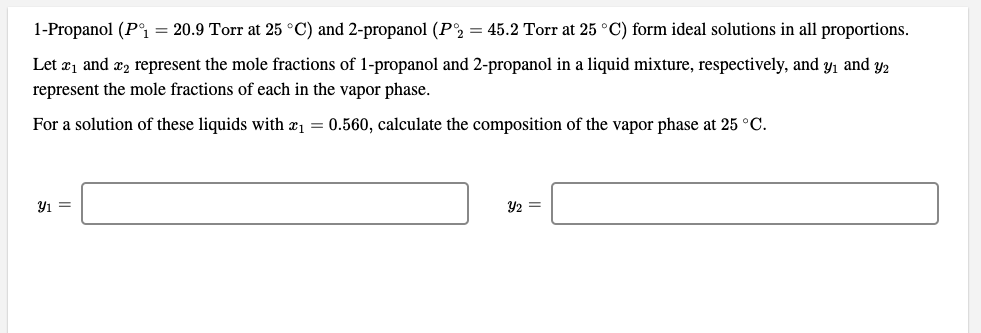help_outlineImage Transcriptionclose1-Propanol (P°i = 20.9 Torr at 25 °C) and 2-propanol (P2 = 45.2 Torr at 25 °C) form ideal solutions in all proportions. Let æ1 and æ2 represent the mole fractions of 1-propanol and 2-propanol in a liquid mixture, respectively, and y, and y2 represent the mole fractions of each in the vapor phase. For a solution of these liquids with æ1 = 0.560, calculate the composition of the vapor phase at 25 °C. Y2 = fullscreen
check_circle

Step 1

According to Raoult’s law :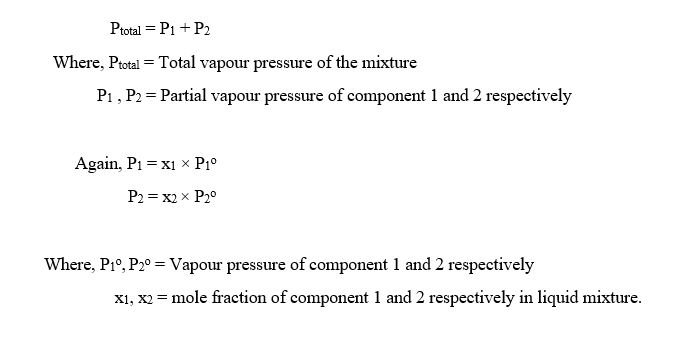Step 2

Given,

Vapour pressure of 1-propanol (component 1), P1o = 20.9 torr

Vapour pressure of 2-propanol (component 2), P2o = 45.2 torr

Mole fraction of 1-propanol, x1 = 0.560

The mole fraction of 2-propanol, x2 can be calculated as: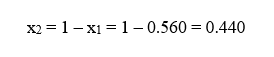Step 3

Partial vapour pressure of 1-propanol and 2-propanol can be calculated as: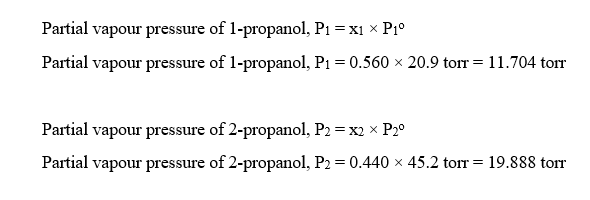The vapour pressure of the mixture can be calculated as :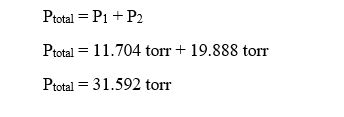...

### Want to see the full answer?

See Solution

#### Want to see this answer and more?

Solutions are written by subject experts who are available 24/7. Questions are typically answered within 1 hour.*

See Solution
*Response times may vary by subject and question.
Tagged in